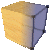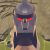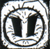## Welcome to the Second Life Forums Archive

These forums are CLOSED. Please visit the new forums HERE

# Discussion: Touch Position on Prims

Jeffrey Gomez
Cubed™Join date: 11 Jun 2004
Posts: 3,522
03-14-2005 17:56
Technically Part Two, Milestone Three of my PrimPorter Project. I figured everyone's getting sick of the headings I keep adding ad nauseam.

This little script, which is placed in touch_start (see below), returns the relative position a user touches a cube in mouselook, at any rotation. Alternately, it returns the rotated bounding box to any other prim (but not object).

CODE
`default{    state_entry()    {        llSetStatus(STATUS_BLOCK_GRAB,TRUE);    }    touch_start(integer total_number)    {        list spawns = [];                vector start = llDetectedPos(0);        vector height = llGetAgentSize(llDetectedKey(0));        start += (<0,0,0.85 * height.z> / 2);         vector diff = llRot2Fwd(llDetectedRot(0));                vector mypos = llGetPos();        rotation myrot = llGetRot();        vector size = llGetScale();        size /= 2;                // Unrotate our vectors...        diff /= myrot;        start = mypos + ((start - mypos) / myrot);                 if(diff.x == 0) diff.x = 0.01;        if(diff.y == 0) diff.y = 0.01;        if(diff.z == 0) diff.z = 0.01;                vector zero_x = start + ((mypos.x - start.x) * diff / diff.x) - mypos;        // When X = 0        vector zero_y = start + ((mypos.y - start.y) * diff / diff.y) - mypos;        // When Y = 0        vector zero_z = start + ((mypos.z - start.z) * diff / diff.z) - mypos;        // When Z = 0                 vector temp;        // Plane: Face 0        temp = zero_z + (size.z * diff / diff.z);        if(llSqrt(llPow(temp.x,2)) <= size.x && llSqrt(llPow(temp.y,2)) <= size.y)        {            spawns += temp;            spawns += 0;        }                        // Plane: Face 1        temp = zero_y - (size.y * diff / diff.y);        if(llSqrt(llPow(temp.x,2)) <= size.x && llSqrt(llPow(temp.z,2)) <= size.z)        {            spawns += temp;            spawns += 1;        }                    // Plane: Face 2        temp = zero_x + (size.x * diff / diff.x);        if(llSqrt(llPow(temp.y,2)) <= size.y && llSqrt(llPow(temp.z,2)) <= size.z)        {            spawns += temp;            spawns += 2;        }                    // Plane: Face 3        temp = zero_y + (size.y * diff / diff.y);        if(llSqrt(llPow(temp.x,2)) <= size.x && llSqrt(llPow(temp.z,2)) <= size.z)        {            spawns += temp;            spawns += 3;        }                    // Plane: Face 4        temp = zero_x - (size.x * diff / diff.x);        if(llSqrt(llPow(temp.y,2)) <= size.y && llSqrt(llPow(temp.z,2)) <= size.z)        {            spawns += temp;            spawns += 4;        }                    // Plane: Face 5        temp = zero_z - (size.z * diff / diff.z);        if(llSqrt(llPow(temp.x,2)) <= size.x && llSqrt(llPow(temp.y,2)) <= size.y)        {            spawns += temp;            spawns += 5;        }                    integer i;        integer count = llGetListLength(spawns);                            count = llGetListLength(spawns);        for(i = 0; i < count; i += 2)        {            temp = llList2Vector(spawns,i);            temp = <temp.x / size.x,temp.y / size.y, temp.z / size.z>;                        llSay(0,"Intersection at Face " + (string)llList2Integer(spawns,i + 1) + " at " + (string)temp);        }    }}`
_____________________
---
Huns Valen
Don't PM me here.Join date: 3 May 2003
Posts: 2,749
Post jack to link original thread
03-16-2005 10:32
http://forums.secondlife.com/showthread.php?t=38717

----------
Now back to your regularly scheduled post, ne
----------

This is not guaranteed to be accurate since you have to guess at the eye height of the agent (due to varying torso:leg height ratios.) In order to make it accurate, it is necessary to calibrate it beforehand. Xylor did a "laser pointer" a while back and I think it worked by having the agent click on a point at the center of the screen. I have a script that does something similar.

The code I use is this:

vector avAngle = llRot2Fwd(detectedRot);
float theta = (llAsin(avAngle.z)) * RAD_TO_DEG;
heightAdjust = distEyeToSurface * llTan(theta * DEG_TO_RAD);

This code assumes that the agent is pointed due east, looking at a surface that is exactly vertical. The degrees/radians conversions are primarily so I can debug the angle. I think you can take them out with no ill effect.

distToEyeSurface is the length of the adjacent leg of the triangle. It is the horizontal distance between the eye and the plane of the surface, with the eye at zero degrees of pitch. The surface itself may not intersect the center ray of the eye - the ray just has to intersect the plane, not necessarily the surface itself.

heightAdjust is a pretty good approximation but there will be small variances as the avatar's head tends to sway back and forth even while not in motion.
_____________________
blaze Spinnaker
1/2 Serious
Join date: 12 Aug 2004
Posts: 5,898
03-16-2005 11:35
Why mouselook?
_____________________
Taken from The last paragraph on pg. 16 of Cory Ondrejka's paper "Changing Realities: User Creation, Communication, and Innovation in Digital Worlds :

"User-created content takes the idea of leveraging player opinions a step further by allowing them to effectively prototype new ideas and features. Developers can then measure which new concepts most improve the products and incorporate them into the game in future patches."
Jeffrey Gomez
Cubed™Join date: 11 Jun 2004
Posts: 3,522
03-16-2005 14:10
Thanks for flagging that, Huns - it's a valid point to make. It's also of relevance that this system is a working example for standing, static avatars - I've not yet toyed with sitting, animations... etc.

As for blaze's question: When an avatar is not in mouselook, calling that avatar's "rotation" returns the avatar's root rotation as opposed to "where that avatar is looking." This is also why most manual gun scripts require avatars to be in mouselook to be used.

Edit: Nope, Lance. It detects where the avatar's field of vision pierces a plane, then reports where.
_____________________
---
Lance LeFay
is a ThugJoin date: 1 May 2003
Posts: 1,488
03-16-2005 19:51
Wouldn't this only work if the av was aiming at the center?
_____________________
"Hoochie Hair is high on my list" - Andrew Linden
"Adorable is 'they pay me to say you are cute'" -Barnesworth Anubis
Zepp Zaftig
Unregistered Abuser
Join date: 20 Mar 2005
Posts: 470
01-15-2006 09:58
vector start = llGetCameraPos();
vector diff = llRot2Fwd(llGetCameraRot());

This should make it work while sitting etc.